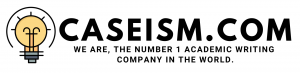# Lab International Inc. Case Solution & Answer

## Lab International Inc. Case Solution

Selling off LAB Pharma Division

Merits

With the historical analysis, it can be seen that this division. due to its nature, is yet to show any profitÂ  for the business and is just involved in the consumption of cash, which it acquires from the other division. Moreover, selling this particular division could help the combined company to increase its worth in the eyes of the market because of non-performing. Last but not the least, the fund required by the Lab Research Division could be adjusted with the sale of LAB Pharma division.

Demerits

The investors have interest in funding the company in future because LAB Pharma admitted the mistake of purchasing Focus Inhalation. The market perception of the less value of the Division will result in a low selling price for the division. It is also challenging and difficult to find a company that has a matching product portfolio to that of the lab.

Valuation of Lab Research Division

In order to value the Lab Research Division in the event of an IPO, the different methods proposed in the case are given below:

• Using the Discounted Cash Flow Method
• Using the Comparable Company Analysis

Discounted Cash Flow Method

Using this method, the value of the division derived would be known as the Intrinsic Value of the company. The case provides sufficient information to perform the DCF valuation. It can be seen that using the value of Beta of 1.30 and the market risk premium of 6.50 (5.00 + 1.50) and the risk free rate of 4.15% (the yield from the five year Canadian Bond), the value of the cost of equity for the company is computed to be 13%, which was computed using the Capital Asset Pricing Model. Moreover, for the value of cost of debt of the company, the interest on the long-term debt is divided by the long-term debt, which derives the value to be 10%. The tax rate for the company given in the case is 32%, which would not subject to any change. For the weights of equity as well as the debt, the carve-out balance sheet for the Lab Research Division is used, which derives the value of weight of equity to be 16% whereas the weight of debt is 84%. Using these values in the underlying formula:

WACC = (Cost of Equity * Weight of Equity) + (Cost of Debt * Weight of Debt) * (1 â€“ Tax Rate)

The weighted average cost of capital is computed to about 8% whereas for the terminal growth rate, it is assumed to be 4%. The free cash flows associated with this division are computed using the following formula:

FCFF = EBIT (1- Tax) + Depreciation â€“ Capital Expenditure â€“ Changes in Working Capital

Then by discounting the future cash flows using the discount rate of 8%, the enterprise value of the company is computed to about \$ 156,375, which is added with cash to get the firm value and it is later subtracted from the debt value obtained from the balance sheet to get the Equity Value, which is computed to about \$ 149,733. The overall computation is shown below:

 Â 2006E 2007E 2008E 2009E 2010E EBITDA 9,000 10,080 11,700 14,250 16,800 Depreciation and amortization 3,500 4,000 4,500 4,600 4,700 EBIT 5,500 6,080 7,200 9,650 12,100 TAX RATE 32% 32% 32% 32% 32% EBIT(1-TAX RATE) 3,740 4,134 4,896 6,562 8,228 Depreciation and amortization 3,500 4,000 4,500 4,600 4,700 OPERATING CASH FLOW 7,240 8,134 9,396 11,162 12,928 LESS: CAPITAL EXPENDITURE 10,000 12,000 12,000 4,000 4,000 LESS: CHANGES IN WORKING CAPITAL 400 576 864 960 864 FREE CASH FLOW -3,160 -4,442 -3,468 6,202 8,064 TERMINAL VALUE 225,821 Net FCF -3,160 -4,442 -3,468 6,202 233,885 ENTERPRISE VALUE 156,375 CASH AND CASH EQUIVALENTS 3,727 Debt 10,369 EQUITY VALUE 149,733

……………………..

This is just a sample partial work. Please place the order on the website to get your own originally done case solution